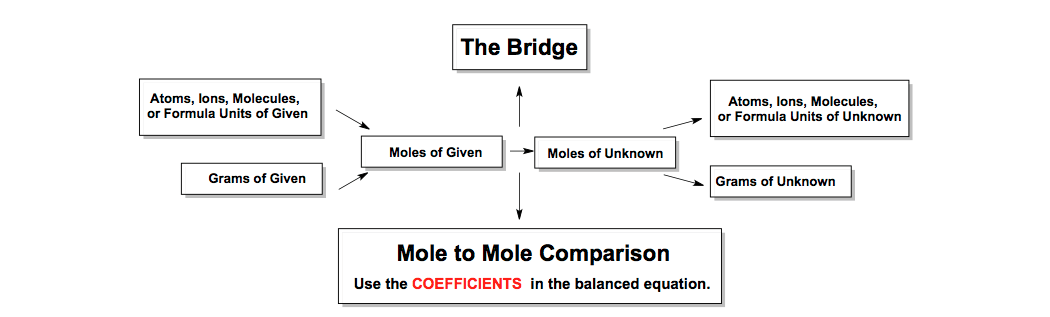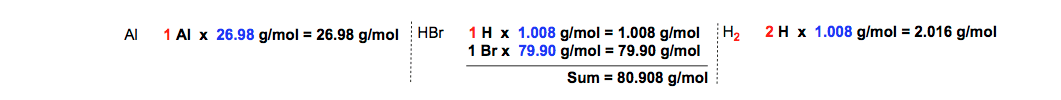Clutch Prep is now a part of Pearson
Ch.3 - Chemical ReactionsWorksheetSee all chapters

# Limiting Reactant (IGNORE)

See all sections
Sections
Empirical Formula
Molecular Formula
Combustion Analysis
Combustion Apparatus
Polyatomic Ions
Naming Ionic Compounds
Writing Ionic Compounds
Naming Ionic Hydrates
Naming Acids
Naming Molecular Compounds
Balancing Chemical Equations
Stoichiometry
Limiting Reagent
Percent Yield
Mass Percent
Functional Groups in ChemistryJules Bruno

The limiting reactant or limiting reagent represents the compound that is totally consumed within a chemical reaction, while the excess reactant represents the compound left over at the end of the chemical process.

The Theoretical Yield

In order to determine which reactant is the limiting reactant we must calculate the theoretical yield, which represents the maximum amount of product that can possibly made.

Stoichiometric Chart

In order to determine the theoretical yield of a reaction you must utilize the stoichiometric chart.How to do Stoichiometry (Stoichiometric Chart)

Calculating Theoretical Yield

In order to calculate the theoretical yield of a chemical reaction we will be given the amounts of our reactants and a balanced chemical equation.

For example, if 21.7 g Al reacts with 34.8 g HBr how many grams of H2 is produced?Al, HBr, AlBr3, H2 Balanced Chemical Equation

STEP 1: Find the atomic masses of the elements from the periodic table.Atomic Masses (Aluminum, hydrogen, bromine)

Do any necessary adding to find the molar masses of Al, HBr and H2Calculating Molar Masses (Al, HBr, H2)

STEP 2: Convert the grams of reactants into moles.Grams to Moles Conversions (Al, HBr)

STEP 3: Convert the moles of reactants to moles of the H2 product by doing mole-to-mole comparisons.Balanced Chemical Equation (Hydrogen Displacement)

During this step we use the coefficients from the balanced equation.Mole to Mole Comparisons (Moles H2)

STEP 4: Convert the moles of H2 into grams of H2Moles to Grams Conversions (Grams H2)

STEP 5: Determine the theoretical yield.

The theoretical yield, sometimes referred to as the 100% yield, represents the smaller amount calculated. The theoretical yield is therefore 0.433 g H2

STEP 6: Determine the limiting reactant and the excess reactant.

The limiting reactant is the compound that gives the smaller amount of product from our calculations, while the excess reactant is the compound that gives the larger amount of product. Therefore HBr is the limiting reactant and Al is the excess reactant.

Not so limiting

Practice problems dealing with the limiting reactant are very common when dealing with stoichiometry. Stoichiometry itself serves as one of the fundamental concepts you’ll constantly use and connects to other concepts such as mass conversionsempirical formula, percent yield, molarity and solution chemistryJules Bruno

Jules felt a void in his life after his English degree from Duke, so he started tutoring in 2007 and got a B.S. in Chemistry from FIU. He’s exceptionally skilled at making concepts dead simple and helping students in covalent bonds of knowledge.